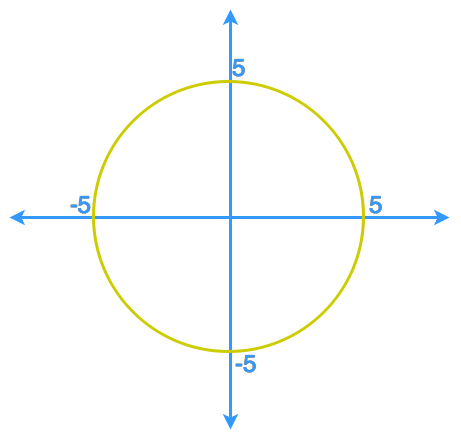# Implicit differentiation

### Implicit differentiation

So far, we have always tried to configure a relation to an explicit function in the form of y = f(x) before finding the derivative of the relation, but what if this is impossible to do so? In this section, we will first learn to identify the difference between explicit functions and implicit functions. Then we will learn how to differentiate a relation with a mix of variables x and y using the method called Implicit Differentiation.

#### Lessons

• Introduction
Explicit Functions VS. Implicit Functions

• 1.
The graph shows a circle centred at the origin with a radius of 5.a) Define the circle implicitly by a relation between x and y .
b) Define the circle by expressing y explicitly in terms of x .
c) Use the method of "explicit differentiation" to find the slope of the tangent line to the circle at the point (4, -3).
d) Use the method of "implicit differentiation" to find the slope of the tangent line to the circle at the point (4, -3).

• 2.
$3{y^4} + 5{x^2}{y^3} - {x^6} = 2x - 9y + 1$
Use implicit differentiation to find: $\frac{{{d}y}}{{{d}x}}$